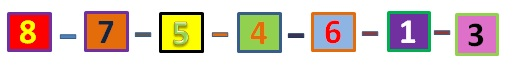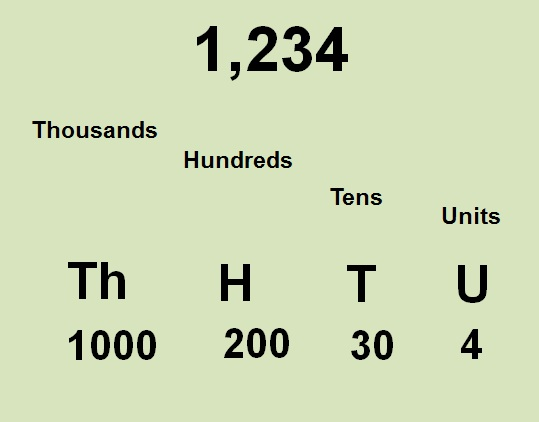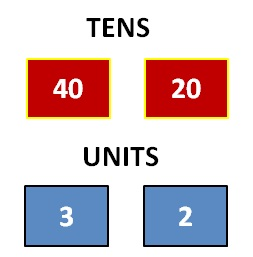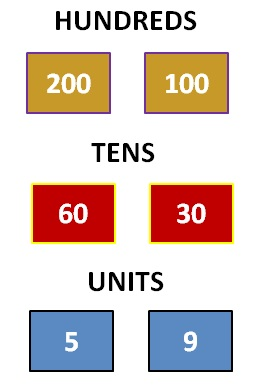# SubractionLook at the examples below before you attempt any of the exercises. It is important to understand how numbers are placed into units, tens, hundreds and thousands.

## Example 1

23 - 6 =?

1. To take 3 away from 6 you will need to borrow from the tens column
2. 13 - 6 = 7 enter 7 in the units column
3. 1 - 0 = 1 enter 1 in the tens column

ThHTU
1213
6
17

-

## Example 2

223 - 18 = ?

1. To take 3 away from 8 you will need to borrow from the tens column
2. 13 - 8 = 5 enter 5 in the units column
3. 1 - 1 = 0 enter 0 in the tens column
4. 2 - 0 = 2 enter 2 in the hundreds column

ThHTU
21213
18
205

-

## Example 3:

1262 - 125 = ?

1. To take 2 away from 5 you will need to borrow from the tens column

2. 12 - 5 = 7 enter 7 in the units column

3. 5 - 2 = 3 enter 3 in the tens column
4. 2 - 1 = 1 enter 1 in the hundreds column
5. 1 - 0 = 1 enter 1 in the thousands column

ThHTU
125612
125
1137

-

# VIDEO

Have a look at the demonstration on the video to enhance your learning.## Example 1:

43 - 22

1. Take the large value 43 and subtract only the tens from the second value 22.
43 - 20 = 23

2. From the value left over 23 subtract the units of the second value, which was 2.
23 - 2 = 21

Total= 21## Example 2:

265 - 139

1. Subtract the hundereds 200 - 100 = 100
2. Subtract 65 - 30 = 35
3. Subtract 35 - 9 = 26

Total = 126Now try the Exercises!